Rolle's and Mean Value Theorem

Chapter 5 Class 12 Continuity and Differentiability
Serial order wise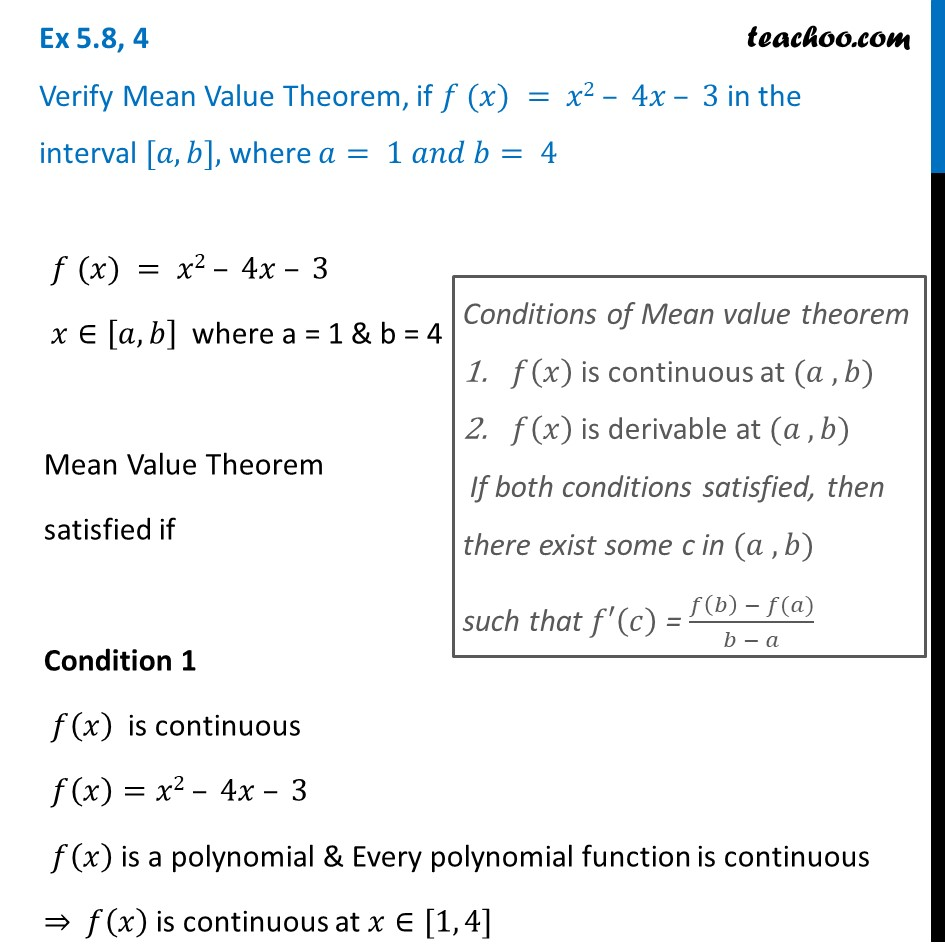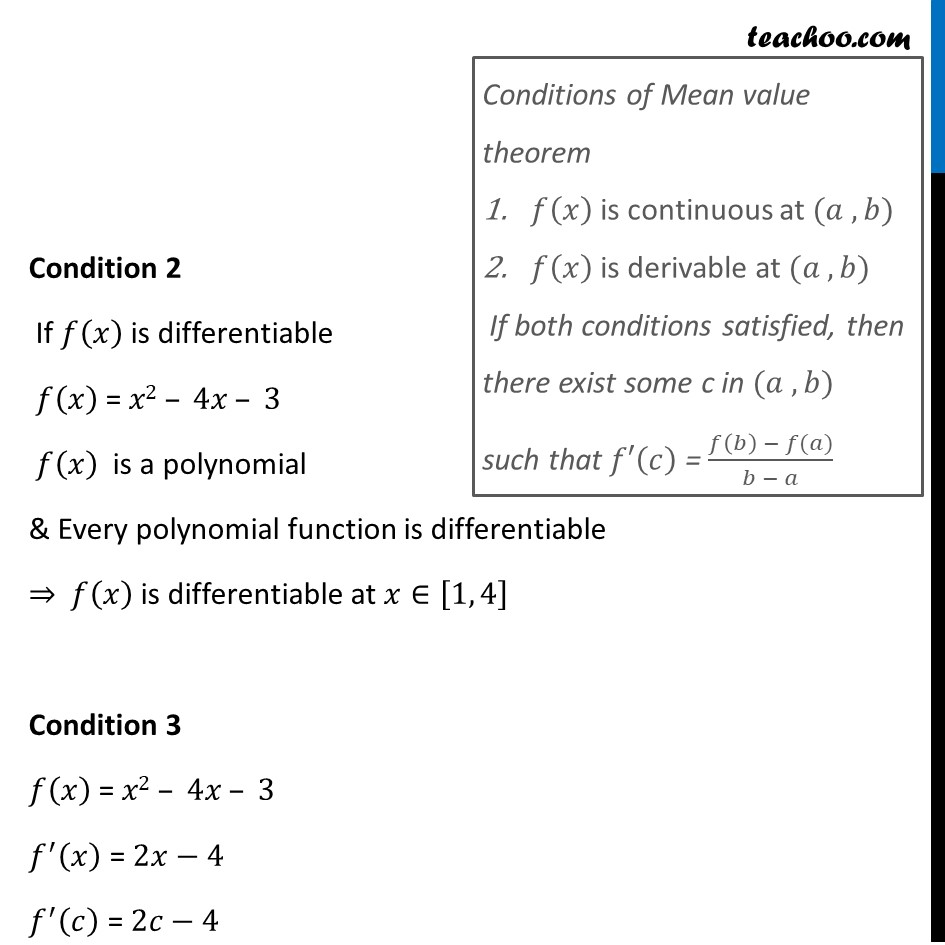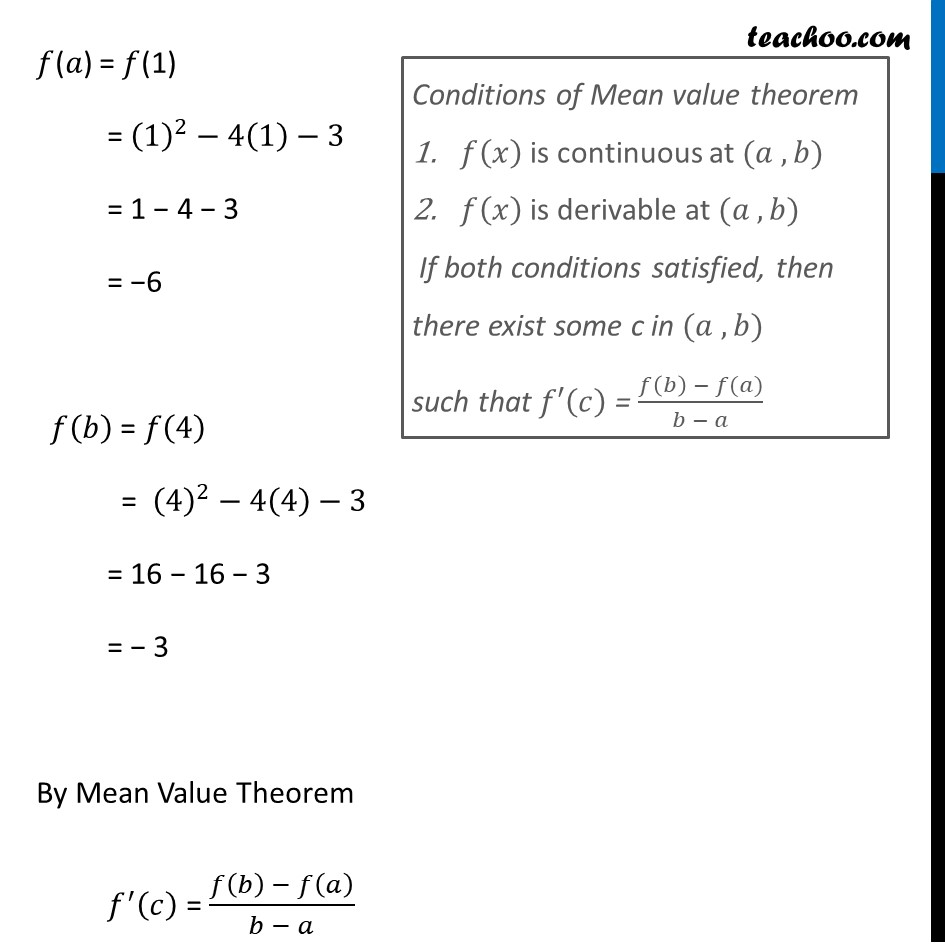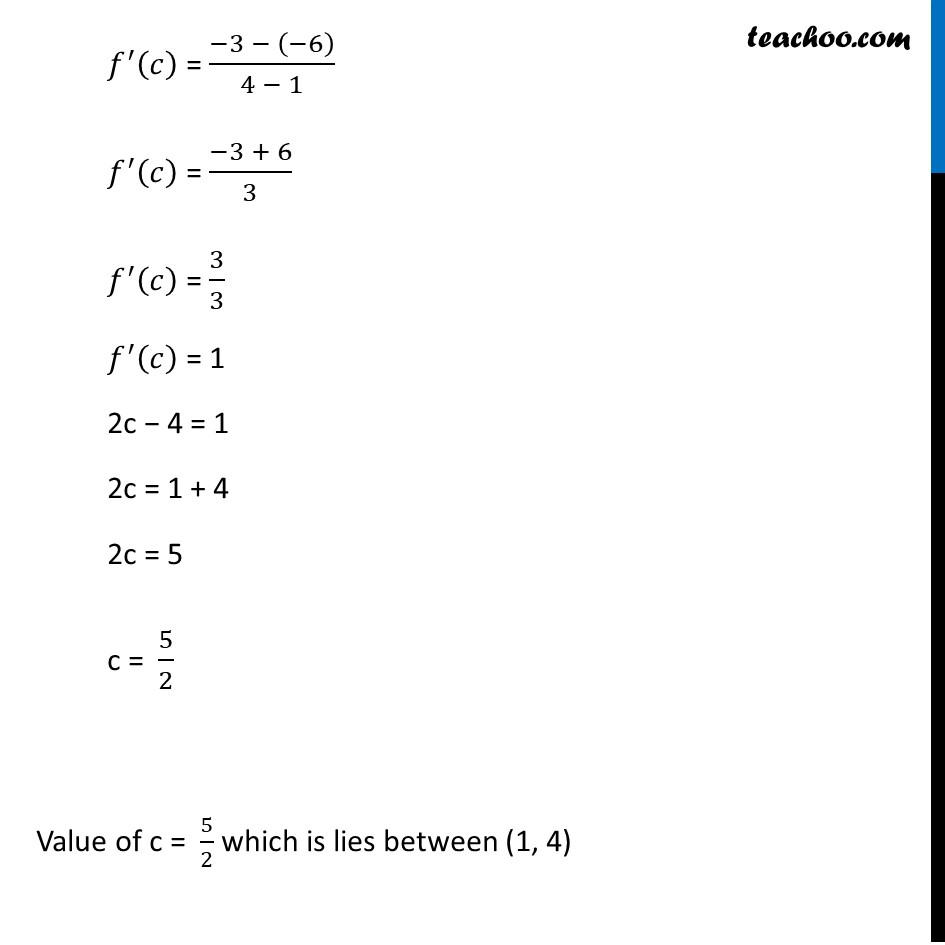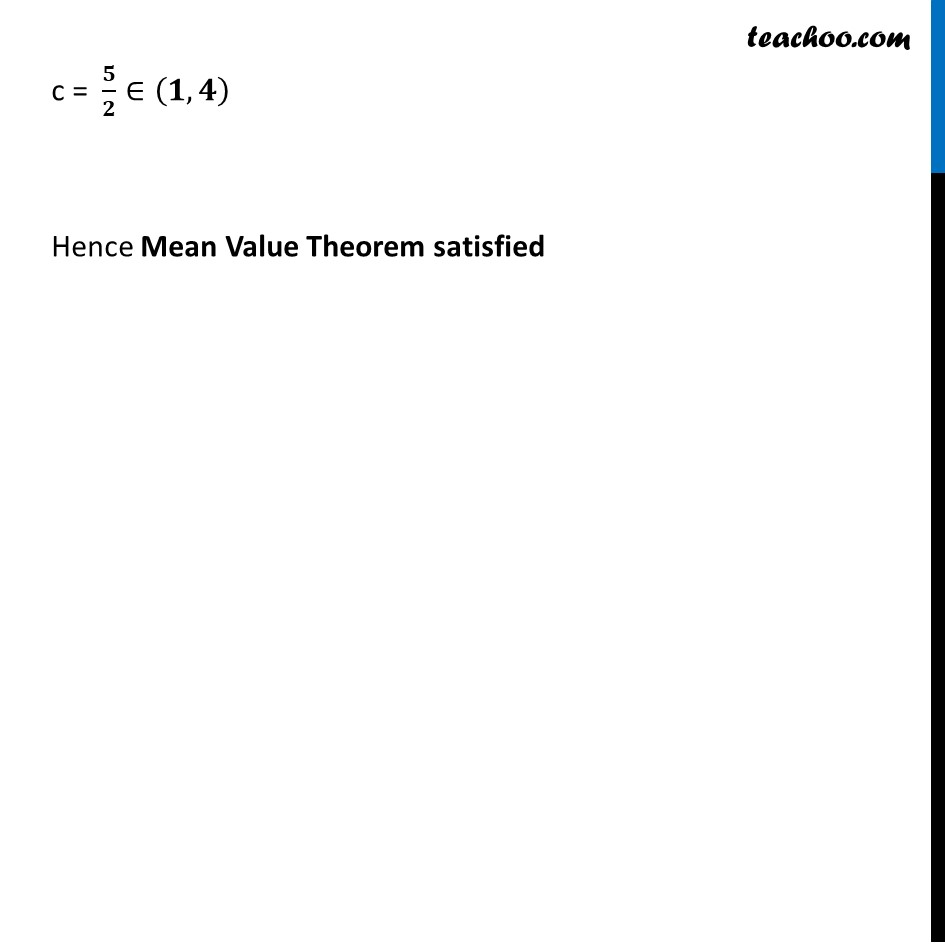Learn in your speed, with individual attention - Teachoo Maths 1-on-1 Class

### Transcript

Question 4 Verify Mean Value Theorem, if 𝑓 (𝑥) = 𝑥2 – 4𝑥 – 3 in the interval [𝑎, 𝑏], where 𝑎= 1 𝑎𝑛𝑑 𝑏= 4 𝑓 (𝑥) = 𝑥2 – 4𝑥 – 3 𝑥∈[𝑎, 𝑏] where a = 1 & b = 4 Mean Value Theorem satisfied if Condition 1 𝑓(𝑥) is continuous 𝑓(𝑥)=𝑥2 – 4𝑥 – 3 𝑓(𝑥) is a polynomial & Every polynomial function is continuous ⇒ 𝑓(𝑥) is continuous at 𝑥∈[1, 4] Conditions of Mean value theorem 𝑓(𝑥) is continuous at (𝑎 , 𝑏) 𝑓(𝑥) is derivable at (𝑎 , 𝑏) If both conditions satisfied, then there exist some c in (𝑎 , 𝑏) such that 𝑓′(𝑐) = (𝑓(𝑏) − 𝑓(𝑎))/(𝑏 − 𝑎) Conditions of Mean value theorem 𝑓(𝑥) is continuous at (𝑎 , 𝑏) 𝑓(𝑥) is derivable at (𝑎 , 𝑏) If both conditions satisfied, then there exist some c in (𝑎 , 𝑏) such that 𝑓′(𝑐) = (𝑓(𝑏) − 𝑓(𝑎))/(𝑏 − 𝑎) Condition 2 If 𝑓(𝑥) is differentiable 𝑓(𝑥) = 𝑥2 – 4𝑥 – 3 𝑓(𝑥) is a polynomial & Every polynomial function is differentiable ⇒ 𝑓(𝑥) is differentiable at 𝑥∈[1, 4] Condition 3 𝑓(𝑥) = 𝑥2 – 4𝑥 – 3 𝑓^′ (𝑥) = 2𝑥−4 𝑓^′ (𝑐) = 2𝑐−4 Conditions of Mean value theorem 𝑓(𝑥) is continuous at (𝑎 , 𝑏) 𝑓(𝑥) is derivable at (𝑎 , 𝑏) If both conditions satisfied, then there exist some c in (𝑎 , 𝑏) such that 𝑓′(𝑐) = (𝑓(𝑏) − 𝑓(𝑎))/(𝑏 − 𝑎) 𝑓(𝑎) = 𝑓(1) = (1)^2−4(1)−3 = 1 − 4 − 3 = −6 𝑓(𝑏) = 𝑓(4) = (4)^2−4(4)−3 = 16 − 16 − 3 = − 3 By Mean Value Theorem 𝑓^′ (𝑐) = (𝑓(𝑏) − 𝑓(𝑎))/(𝑏 − 𝑎) 𝑓^′ (𝑐) = (−3 − (−6))/(4 − 1) 𝑓^′ (𝑐) = (−3 + 6)/3 𝑓^′ (𝑐) = 3/3 𝑓^′ (𝑐) = 1 2c − 4 = 1 2c = 1 + 4 2c = 5 c = 5/2 Value of c = 5/2 which is lies between (1, 4) c = 𝟓/𝟐∈(𝟏, 𝟒) Hence Mean Value Theorem satisfied